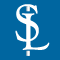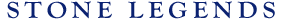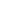HOMEPRODUCTSIDEA CENTERSERVICESTECHNICALABOUT USHello Guest Login  |  Become Member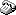800-398-1199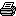214-398-1293MapEmail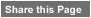Click to Share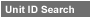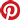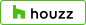Select which variables you would like to search by.Type DescriptionPrimary ViewPrimary ModifierUnit NameView Definitions

View Definitions

View DefinitionsX-Dim Y-Dim Z-Dim XX-Dim YY-Dim ZZ-Dim R-Dim D-Dim P-Dim
 Click column heading to re-sort results list.Product Name Primary View Modifier Pri Dimx  y  z Alt Dimxx  yy  zz Alt2 Dimr  d  p E\$timated Thumbnail Alpine Bolson Table Circular Pedestal X = 48" Y = 31-3/4"Z = 48" XX = n/a YY = n/aZZ = n/a R = n/a D = 48"P = n/a \$ 713.00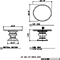Lavalle Table Table DecorativeLegs X = 33-3/4" Y = 40-1/2"Z = 15-1/4" XX = 51-3/4" YY = 43-1/2"ZZ = 8-1/2" R = n/a D = n/aP = n/a \$ 599.00Triste Corinthian Table Table Circular Square X = 48" Y = 30"Z = 48" XX = n/a YY = n/aZZ = n/a R = n/a D = n/aP = n/a \$ 738.00Triste Soldana Table Table Circular X = 48" Y = 31-1/2"Z = 48" XX = n/a YY = n/aZZ = n/a R = n/a D = 48"P = n/a \$ 1200.00Triste Table Leg Table DecorativeLegs X = 7" Y = 28-1/4"Z = 18" XX = n/a YY = n/aZZ = n/a R = n/a D = n/aP = n/a \$ 320.67Triste Table Top Table Circular X = 48" Y = 48"Z = 3-1/4" XX = n/a YY = n/aZZ = n/a R = 24" D = 48"P = n/a \$ 238.00